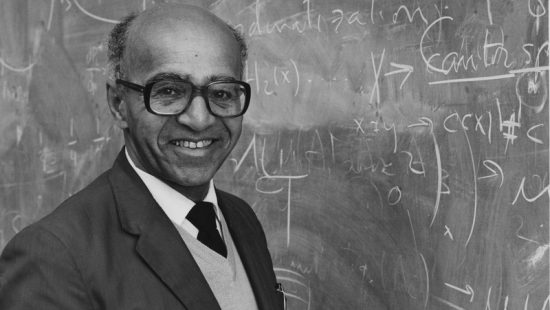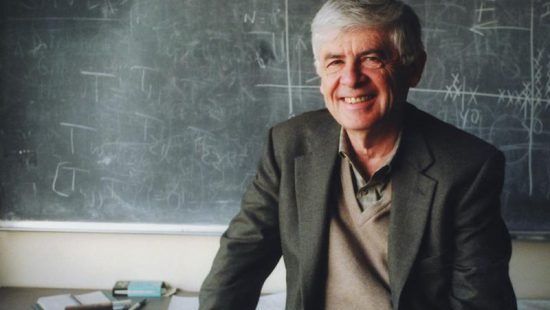# Richard M. Karp

• National Medal of Science
• Mathematics And Computer Science

For his pioneering research in theoretical computer science and the development of NP-Completeness, a concept having an important role in the theory and the practice of computation.

Richard M. Karp began his career as a computer scientist and computational theorist at IBM’s Thomas J. Watson Research Center. In 1968, he became Professor of Computer Science, Mathematics, and Operations Research at the University of California, Berkeley. Karp is the founding Director of the Simons Institute for the Theory of Computing at Berkeley.

The unifying theme in Karp’s work has been the study of combinatorial algorithms. His 1972 paper, “Reducibility Among Combinatorial Problems,” showed that many of the most commonly studied combinatorial problems are NP-complete, and hence likely to be intractable. Much of his work has concerned parallel algorithms, the probabilistic analysis of combinatorial optimization algorithms and the construction of randomized algorithms for combinatorial problems.

Karp’s work on NP-completeness motivated the discussion of the unsolved P = NP question, a question considered one of the most important open questions in theoretical computer science and mathematics. P = NP asks whether finding a solution to a problem is inherently more difficult than verifying that a proposed solution is correct. Karp introduced the now standard methodology for proving problems to be NP-complete, leading to the identification of many theoretical and practical problems as being computationally difficult.

By Jen Santisi

## Related Laureates### Michael Artin### David Blackwell